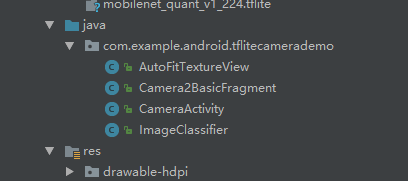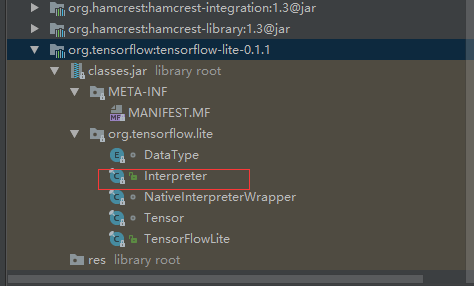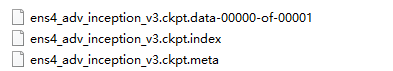# Tensorflow Lite初探（Android）

## 一、背景：

11月15日，谷歌正式发布了TensorFlow Lite开发者预览版。

TensorFlow Lite 是 Google I/O 2017 大会上的其中一个重要宣布，有了TensorFlow Lite，应用开发者可以在移动设备上部署人工智能。

Google 表示 Lite 版本 TensorFlow 是 TensorFlow Mobile 的一个延伸版本。尽管是一个轻量级版本，依然是在智能手机和嵌入式设备上部署深度学习的一大动作。此前，通过TensorFlow Mobile API，TensorFlow已经支持手机上的模型嵌入式部署。TensorFlow Lite应该被视为TensorFlow Mobile的升级版。

TensorFlow Lite 目前仍处于“积极开发”状态，目前仅有少量预训练AI模型面世，比如MobileNet、用于计算机视觉物体识别的Inception v3、用于自然语言处理的Smart Reply，当然，TensorFlow Lite上也可以部署用自己的数据集定制化训练的模型。

TensorFlow Lite可以与Android 8.1中发布的神经网络API完美配合，即便在没有硬件加速时也能调用CPU处理，确保模型在不同设备上的运行。 而Android端版本演进的控制权是掌握在谷歌手中的，从长期看，TensorFlow Lite会得到Android系统层面上的支持。• TensorFlow 模型（TensorFlow Model）：保存在磁盘中的训练模型。
• TensorFlow Lite 转化器（TensorFlow Lite Converter）：将模型转换成 TensorFlow Lite 文件格式的项目。
• TensorFlow Lite 模型文件（TensorFlow Lite Model File）：基于 FlatBuffers，适配最大速度和最小规模的模型。

## 二、环境：

Android Studio 3.0, SDK Version API26, NDK Version 14

1. 将此项目导入到Android Studio：
https://github.com/tensorflow/tensorflow/tree/master/tensorflow/contrib/lite/java/demo
2. 下载移动端的模型（model）和标签数据（lables）：
https://storage.googleapis.com/download.tensorflow.org/models/tflite/mobilenet_v1_224_android_quant_2017_11_08.zip
3. 下载完成解压mobilenet_v1_224_android_quant_2017_11_08.zip文件得到一个xxx.tflite和labes.txt文件，分别是模型和标签文件，并且把这两个文件复制到assets文件夹下。
4. 构建app，run……

## 三、源码分析：- AutoFitTextureView: 一个自定义View；
- CameraActivity: 整个app的入口activity，这个activity只做了一件事，就是加载了一个fragment；
- Camera2BasicFragment: 入口activity中加载的fragment，其中实现了所有跟UI相关的代码；首先在onActivityCreated中，初始化了一个ImageClassifier对象，此类是整个demo的核心，用于加载模型并实现推理运算功能。然后开启了一个后台线程，在线程中反复地对从摄像头获取的图像进行分类操作。

/** Load the model and labels. */
@Override
public void onActivityCreated(Bundle savedInstanceState) {
super.onActivityCreated(savedInstanceState);
try {
classifier = new ImageClassifier(getActivity());
} catch (IOException e) {
Log.e(TAG, "Failed to initialize an image classifier.");
}
startBackgroundThread();
}

startBackgroundThread()中做的轮询操作：

private Runnable periodicClassify =
new Runnable() {
@Override
public void run() {
synchronized (lock) {
if (runClassifier) {
classifyFrame();
}
}
backgroundHandler.post(periodicClassify);
}
};

/** Classifies a frame from the preview stream. */
private void classifyFrame() {
if (classifier == null || getActivity() == null || cameraDevice == null) {
showToast("Uninitialized Classifier or invalid context.");
return;
}
Bitmap bitmap =
textureView.getBitmap(ImageClassifier.DIM_IMG_SIZE_X, ImageClassifier.DIM_IMG_SIZE_Y);
String textToShow = classifier.classifyFrame(bitmap);
bitmap.recycle();
showToast(textToShow);
}

ImageClassifier：demo最重要的部分，但只有两个函数比较重要，一个是构造函数：

/** Initializes an {@code ImageClassifier}. */
ImageClassifier(Activity activity) throws IOException {
tflite = new Interpreter(loadModelFile(activity));
labelList = loadLabelList(activity);
imgData =
ByteBuffer.allocateDirect(
DIM_BATCH_SIZE * DIM_IMG_SIZE_X * DIM_IMG_SIZE_Y * DIM_PIXEL_SIZE);
imgData.order(ByteOrder.nativeOrder());
labelProbArray = new byte[labelList.size()];
Log.d(TAG, "Created a Tensorflow Lite Image Classifier.");
}tflite = new Interpreter(loadModelFile(activity))这里通过loadModelFile将asset中的tflite格式的模型文件加载并返回一个MappedByteBuffer传给Interpreter。labelList = loadLabelList(activity)将asset中的labels文件中的分类标签加载到字符串列表labelList中。imgData则是一个存放输入张量的buffer，一个非常典型的（batch_size, x, y, channel)结构，在这里可以理解为一个placeholder

/** Classifies a frame from the preview stream. */
String classifyFrame(Bitmap bitmap) {
if (tflite == null) {
Log.e(TAG, "Image classifier has not been initialized; Skipped.");
return "Uninitialized Classifier.";
}
convertBitmapToByteBuffer(bitmap);
// Here's where the magic happens!!!
long startTime = SystemClock.uptimeMillis();
tflite.run(imgData, labelProbArray);
long endTime = SystemClock.uptimeMillis();
Log.d(TAG, "Timecost to run model inference: " + Long.toString(endTime - startTime));
String textToShow = printTopKLabels();
textToShow = Long.toString(endTime - startTime) + "ms" + textToShow;
return textToShow;
}

#对于这行代码，有没有似曾相识的感觉：
tf.Session().run(output, feed_dict={x:input})

## 四、关于tflite模型

https://github.com/tensorflow/tensorflow/tree/master/tensorflow/contrib/lite

import tensorflow as tf
img = tf.placeholder(name="img", dtype=tf.float32, shape=(1, 64, 64, 3))
val = img + tf.constant([1., 2., 3.]) + tf.constant([1., 4., 4.])
out = tf.identity(val, name="out")
with tf.Session() as sess:
tflite_model = tf.contrib.lite.toco_convert(sess.graph_def, [img], [out])
open("converteds_model.tflite", "wb").write(tflite_model)

https://github.com/tensorflow/modelsimport tensorflow as tf

...

saver = tf.train.Saver()
with tf.Session() as sess:
saver.save(sess, "/model/xxxx.ckpt") #在session中将计算图和变量信息保存到ckpt文件中

• GraphDef (.pb) - a protobuf that represents the TensorFlow training and or computation graph. This contains operators, tensors, and variables definitions.
• FrozenGraphDef - a subclass of GraphDef that contains no variables. A GraphDef can be converted to a frozen graphdef by taking a checkpoint and a graphdef and converting every variable into a constant with the value looked up in the checkpoint.

tf.train.write_graph()

https://github.com/tensorflow/tensorflow/tree/master/tensorflow/examples/android

bazel build tensorflow/contrib/lite/toco:toco

bazel-bin/tensorflow/contrib/lite/toco/toco -- \
--input_file=\$(pwd)/mobilenet_v1_1.0_224/frozen_graph.pb \
--input_format=TENSORFLOW_GRAPHDEF  --output_format=TFLITE \
--output_file=/tmp/mobilenet_v1_1.0_224.lite --inference_type=FLOAT \
--input_type=FLOAT --input_arrays=input \
--output_arrays=MobilenetV1/Predictions/Reshape_1 --input_shapes=1,224,224,3

bazel build tensorflow/python/tools:freeze_graph

bazel-bin/tensorflow/python/tools/freeze_graph\
--input_graph=/tmp/mobilenet_v1_224.pb \
--input_checkpoint=/tmp/checkpoints/mobilenet-10202.ckpt \
--input_binary=true --output_graph=/tmp/frozen_mobilenet_v1_224.pb \
--output_node_names=MobileNet/Predictions/Reshape_1

https://github.com/tensorflow/tensorflow/tree/master/tensorflow/contrib/lite/g3doc/models.md